## 04.自定义类型：结构体

news/2023/6/7 0:38:49

# 1 结构体的声明

## 1.2 结构的声明

``````struct tag
{
member-list;//成员列表
}variable-list;//变量列表``````

EG:

``````struct Stu
{char name;//名字int age;//年龄char sex;//性别char id;//学号
}; //分号不能丢 ``````

## 1.3 特殊的声明

``````/*struct Stu
{char name;int age;
} s1,s2;*///全局变量,s1和s2是两个结构体变量typedef struct Stu//将复杂类型简单化
{char name;int age;
}Stu;//相当于将struct stu进行了重命名，主函数运用的时候直接用stu即可。struct
{char name;int age;
}s1;//匿名结构类型，直接创建名字struct
{int a;char c;double d;
}* p;//匿名结构类型的指针，创建pint main()
{struct Stu s3, s4;//局部变量Stu s5, s6;return 0;
}``````
``````//匿名结构体类型
struct
{int a;char b;float c;
}x;//x是全局变量，int main创建的是局部变量
struct
{int a;char b;float c;
}a, * p;``````

//在上面代码的基础上，下面的代码合法吗？
p = &x;

## 1.4 结构的自引用

//链表：

//数据结构->数据在内存中存储的结构

//顺序数据结构：顺序表

//链表（✳）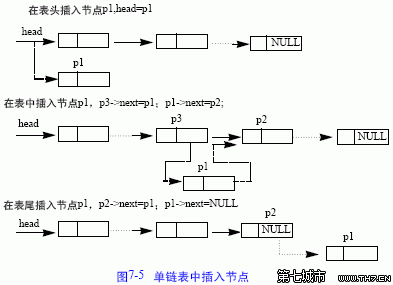//放入同类型的下一个类型的指针（结构体自引用的方式）

//二叉树

``````//代码1
struct Node
{
int data;
struct Node next;
};
//可行否？

``````//代码2
struct Node
{
int data;
struct Node* next;
};``````

``````typedef struct Node
{int data;struct Node* next;
}Node;int main()
{struct Node n1;struct Node n2;n1.next = &n2;return 0;
}``````

注意：

``````/代码3
typedef struct
{
int data;
Node* next;
}Node;
//这样写代码，可行否？
//解决方案：
typedef struct Node
{
int data;
struct Node* next;
}Node;``````

## 1.5 结构体变量的定义和初始化

``````struct Point
{int x;int y;
}p1 = {10, 20};struct Point p2 = {0,0};struct S
{int num;char ch;struct Point p;float d;
};int main()
{struct Point p3 = {1,2};struct S s = { 100, 'w', {2,5}, 3.14f};struct S s2 = {.d=1.2f, .p.x=3,.p.y=5, .ch = 'q', .num=200};printf("%d %c %d %d %f\n", s.num, s.ch, s.p.x, s.p.y, s.d);//乱序初始化printf("%d %c %d %d %f\n", s2.num, s2.ch, s2.p.x, s2.p.y, s2.d);return 0;
}``````

``````struct Point
{
int x;
int y;
}p1; //声明类型的同时定义变量p1
struct Point p2; //定义结构体变量p2
//初始化：定义变量的同时赋初值。
struct Point p3 = {x, y};
struct Stu //类型声明
{
char name;//名字
int age; //年龄
};
struct Stu s = {"zhangsan", 20};//初始化
struct Node
{
int data;
struct Point p;
struct Node* next;
}n1 = {10, {4,5}, NULL}; //结构体嵌套初始化
struct Node n2 = {20, {5, 6}, NULL};//结构体嵌套初始化``````

## 1.6 结构体内存对齐

``````#include <stdio.h>
#include <stddef.h>struct S1
{char c1;//int i;//char c2;//
};struct S2
{char c1;//1char c2;//1int i;//4
};//offsetofstruct S3
{double d;char c;int i;
};struct S4
{char c1;struct S3 s3;double d;
};int main()
{printf("%d\n", sizeof(struct S4));//printf("%d\n", sizeof(struct S3));//printf("%d\n", sizeof(struct S1));//12// 论证方法：宏offsetof//printf("%d\n", sizeof(struct S2));//8//printf("%d\n", offsetof(struct S1, c1));//printf("%d\n", offsetof(struct S1, i));//printf("%d\n", offsetof(struct S1, c2));return 0;
}``````

//S1和S2里面只是改变了顺序，为什么内存大小不一样

1.结构体的第一个成员，对齐到结构体在内存中存放位置的0偏移处

2. 从第二个成员开始，每个成员都要对齐到(一个对齐数) 的整数倍处对齐数: 结构体成员自身大小和默认对齐数的较小值

VS :默认对齐数数8
Linux gcc:没有默认对齐数，对齐数就是结构体成员的自身大小
3.结构体的总大小，必须是所有成员的对文数中最大对齐数的整数倍

4.如果结构体中嵌套了结构体成员，要将嵌套的结构体成员对齐到自己的成员中最大对齐数的整数停处。结构体的总大小必须是最大对齐数的整数倍，这里的最大对齐数是: 包含嵌套结构体成员中的对齐数，的所有对齐数中的最大值。

``````struct S1
{char c1;//最大对齐数：1int i;//最大对齐数：4/8中最小的值，故为4char c2;//最大对齐数：1/4，故为4
};
//现在用了9，必须为最大对齐数4的倍数（又浪费了3个空间），故为12``````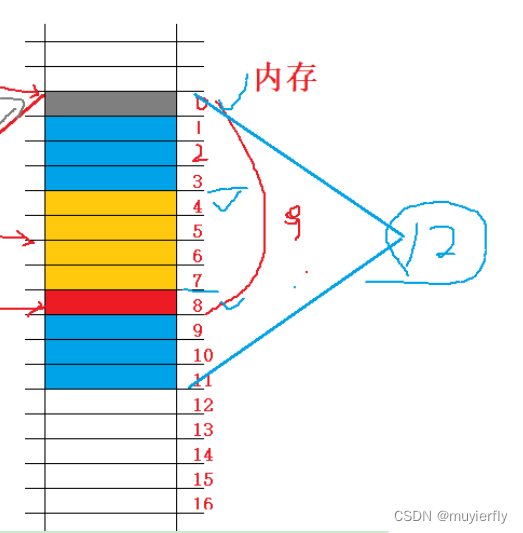``````struct S2
{char c1;//1char c2;//1int i;//4
};``````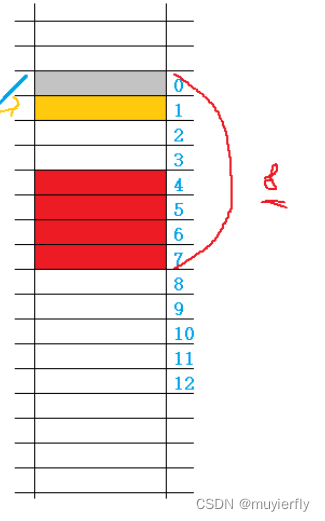s2用到的空间更小，是因为它将小类型的放在一起了

//去较小值为对齐数

``````struct S3
{double d;//占用8个字节 char c;//对齐到整数倍的对齐数（8和char字节的最小值）int i;//4，8最小为4，倍数为12，12，13，14，15
};``````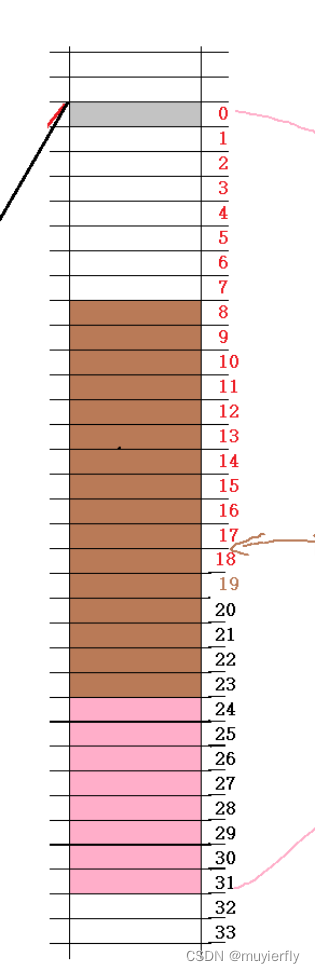1. 平台原因(移植原因)：

2. 性能原因：

``````//例如：
struct S1
{char c1;int i;char c2;
};
struct S2
{char c1;char c2;int i;
};``````

S1和S2类型的成员一模一样，但是S1和S2所占空间的大小有了一些区别。

## 1.7 修改默认对齐数

//VS环境下默认对齐数是8

``````#include <stdio.h>
#pragma pack(8)//设置默认对齐数为8
struct S1
{char c1;int i;char c2;
};
#pragma pack()//取消设置的默认对齐数，还原为默认
#pragma pack(1)//设置默认对齐数为1
struct S2
{char c1;int i;char c2;
};
#pragma pack()//取消设置的默认对齐数，还原为默认
int main()
{//输出的结果是什么？printf("%d\n", sizeof(struct S1));printf("%d\n", sizeof(struct S2));return 0;
}``````

//默认对齐数为1就相当于没有对齐了

## 1.8 结构体传参

``````struct S
{
int data;
int num;
};
struct S s = {{1,2,3,4}, 1000};
//结构体传参
void print1(struct S s)
{
printf("%d\n", s.num);
}
//结构体地址传参
void print2(struct S* ps)
{
printf("%d\n", ps->num);
}
int main()
{
print1(s); //传结构体
print2(&s); //传地址
return 0;
}``````

print2优于print 1

# 2. 位段

## 2.1 什么是位段

1.位段的成员必须是 int、unsigned int 或signed int 。
2.位段的成员名后边有一个冒号和一个数字

EG:

``````struct A
{int _a : 2;int _b : 5;int _c : 10;int _d : 30;
};//节省空间
//作用于结构体类似``````

32:5

2 (30)

5 (25)

10 (15)//有很多的不确定因素

32:

30

A就是一个位段类型。

printf("%d\n", sizeof(struct A));

## 2.2 位段的内存分配

%2.7.02：33：15

//位段是为了节省空间的，不存在对其

1. 位段的成员可以是 int unsigned int signed int 或者是 char （属于整形家族）类型
2. 位段的空间上是按照需要以4个字节（ int ）或者1个字节（ char ）的方式来开辟的。
3. 位段涉及很多不确定因素，位段是不跨平台的，注重可移植的程序应该避免使用位段。

``````//一个例子
struct S
{char a : 3;char b : 4;char c : 5;char d : 4;
};
struct S s = { 0 };
s.a = 10;
s.b = 12;
s.c = 3;
s.d = 4;
//空间是如何开辟的？``````

##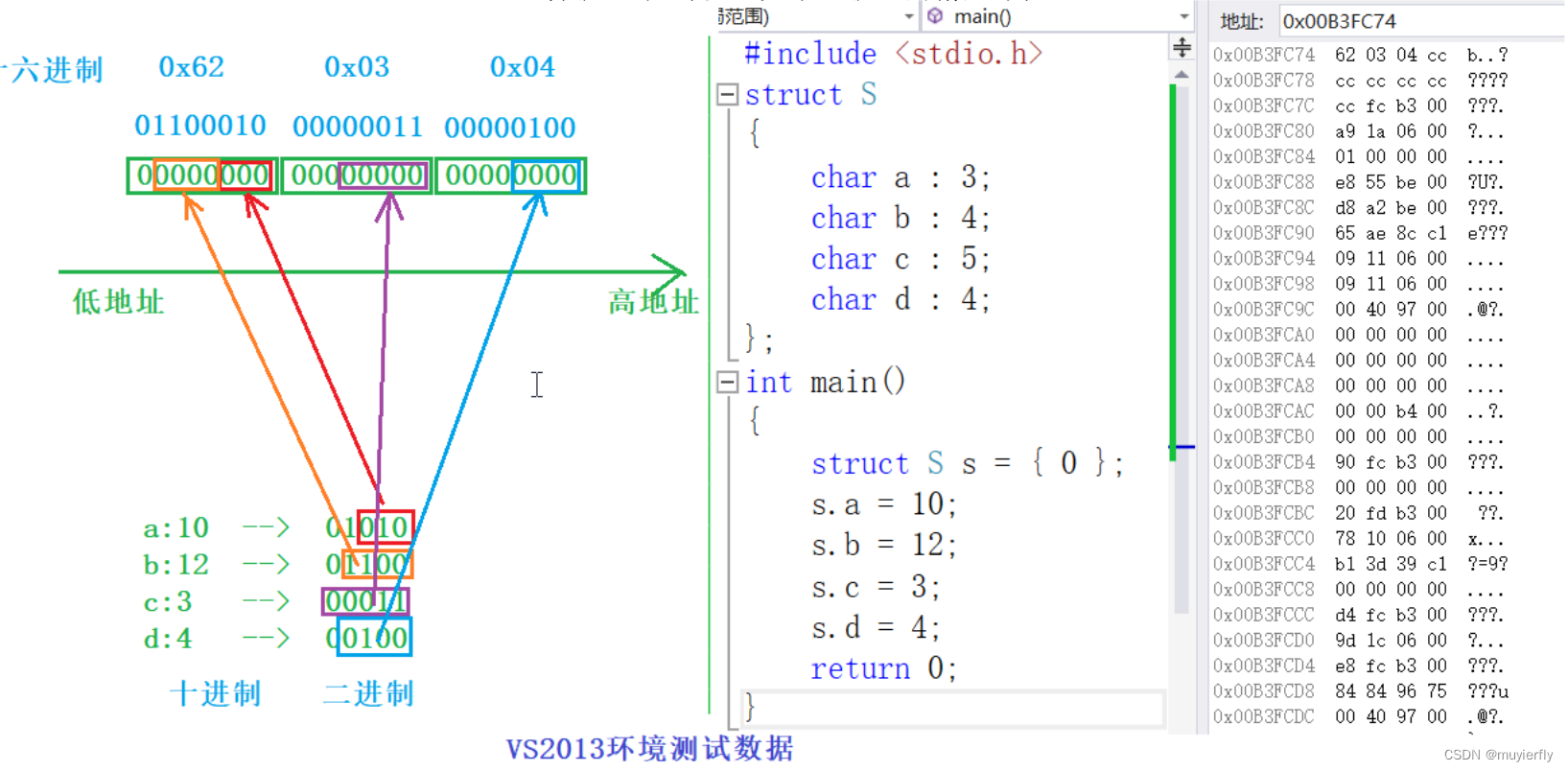2.3 位段的跨平台问题

1. int 位段被当成有符号数还是无符号数是不确定的。
2. 位段中最大位的数目不能确定。（16位机器最大16，32位机器最大32，写成27，在16位机

3. 位段中的成员在内存中从左向右分配，还是从右向左分配标准尚未定义。
4. 当一个结构包含两个位段，第二个位段成员比较大，无法容纳于第一个位段剩余的位时，是舍弃剩余的位还是利用，这是不确定的。

### 2018年文章汇总

Android ANR 实例分析Linux kernel计算某段代码运行时间Linux Kernel 发展和内核特点C/C函数指针与指针函数(二)老王带你理解算法复杂度O(1),O(N),O(N^2)Android NDK Tombstone/Crash 分析堆和栈的区别&#xff08;转过无数次的文章&#xff09;C语言scanf-周末杂想C语言-scanf…